Скачать презентацию Probability and Using a Punnett Square Probability

437548e6aff51df304d60c22adc1beaa.ppt

• Количество слайдов: 17Probability and Using a Punnett Square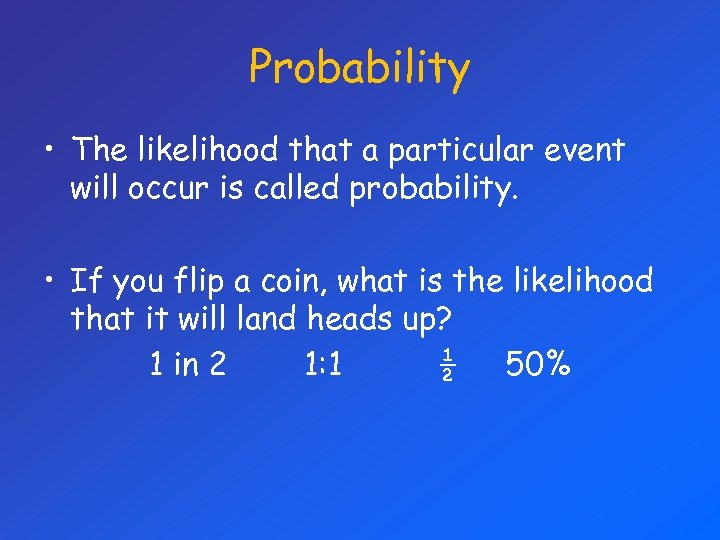Probability • The likelihood that a particular event will occur is called probability. • If you flip a coin, what is the likelihood that it will land heads up? 1 in 2 1: 1 ½ 50%Probability If you flip a coin three times in a row, what is the probability that it will land heads up every time (3 times in a row)? ½ x ½ = 1/8 That the individual probabilities are multiplied together illustrates the point that past outcomes do not affect future outcomes.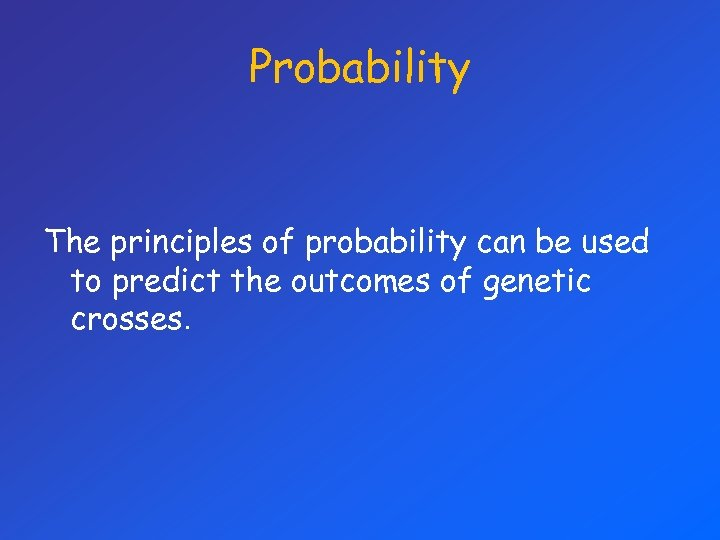Probability The principles of probability can be used to predict the outcomes of genetic crosses.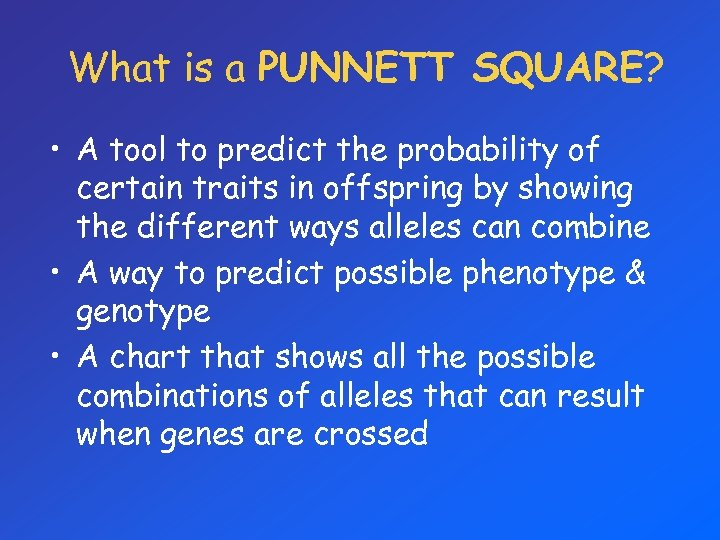What is a PUNNETT SQUARE? • A tool to predict the probability of certain traits in offspring by showing the different ways alleles can combine • A way to predict possible phenotype & genotype • A chart that shows all the possible combinations of alleles that can result when genes are crossed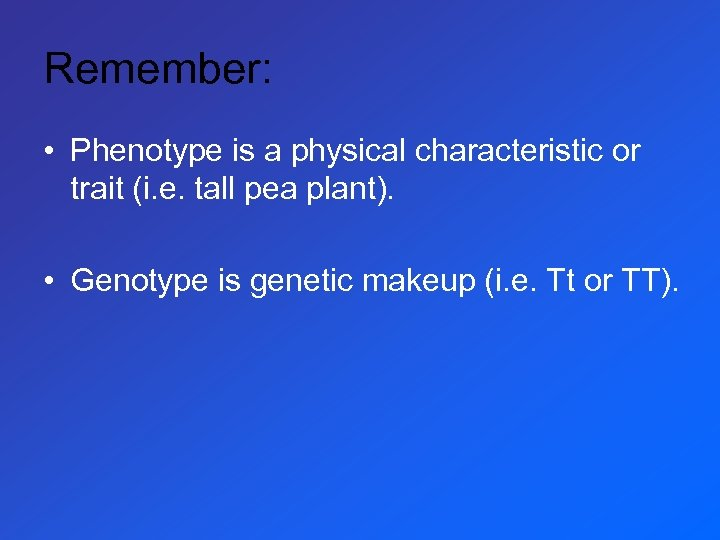Remember: • Phenotype is a physical characteristic or trait (i. e. tall pea plant). • Genotype is genetic makeup (i. e. Tt or TT).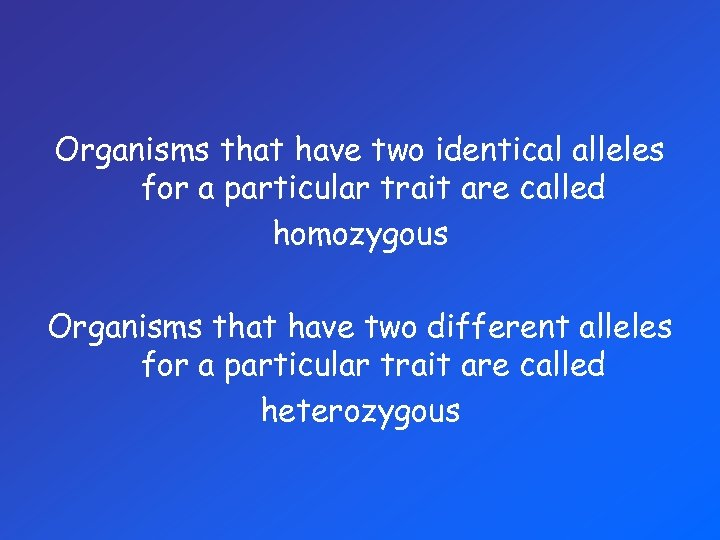Organisms that have two identical alleles for a particular trait are called homozygous Organisms that have two different alleles for a particular trait are called heterozygous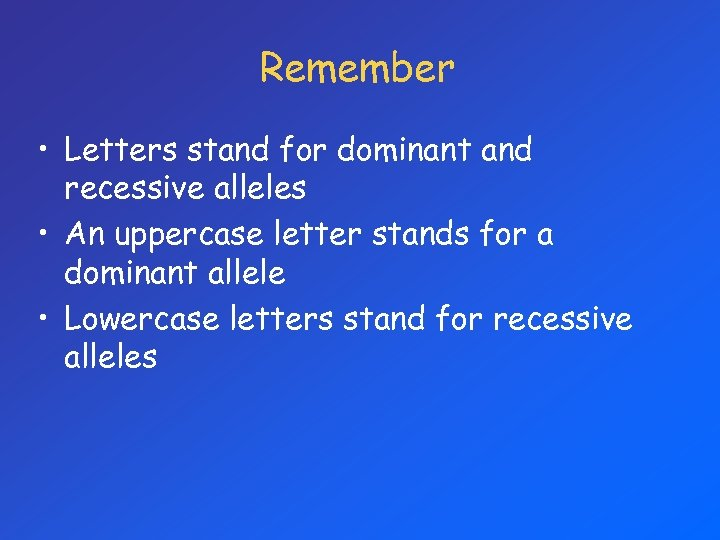Remember • Letters stand for dominant and recessive alleles • An uppercase letter stands for a dominant allele • Lowercase letters stand for recessive allelesUsing a PUNNETT SQUARE To set up a simple Punnett square, draw a large square, and then divide it into 4 equal sections (also squares). It should look something like this: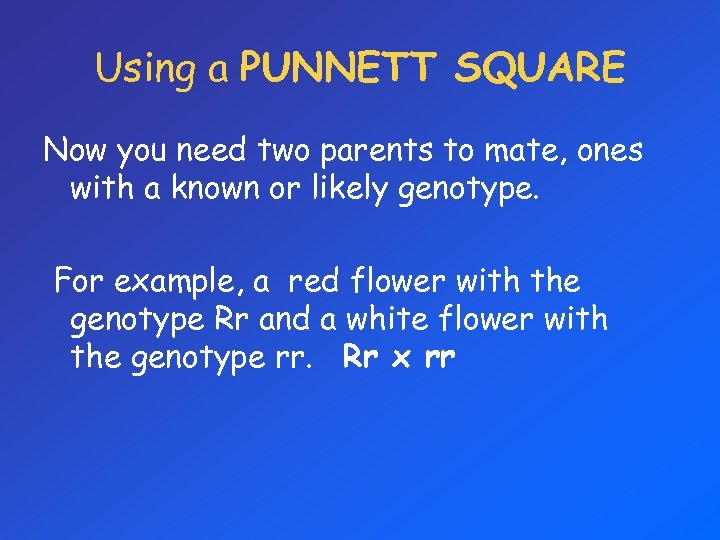Using a PUNNETT SQUARE Now you need two parents to mate, ones with a known or likely genotype. For example, a red flower with the genotype Rr and a white flower with the genotype rr. Rr x rrUsing a PUNNETT SQUARE Place one of the parents on top, and one on the left. You should get something similar to this: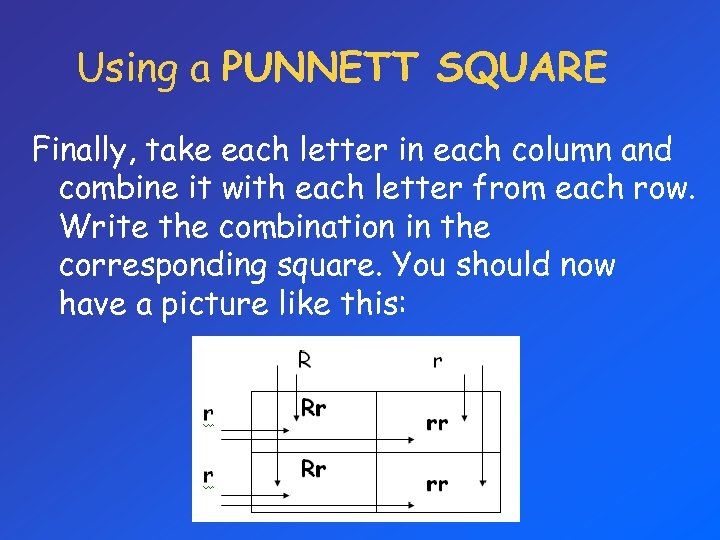Using a PUNNETT SQUARE Finally, take each letter in each column and combine it with each letter from each row. Write the combination in the corresponding square. You should now have a picture like this: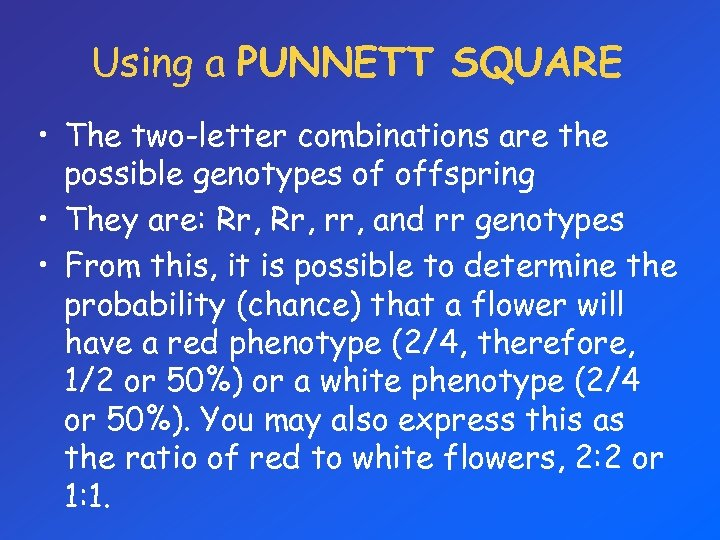Using a PUNNETT SQUARE • The two-letter combinations are the possible genotypes of offspring • They are: Rr, rr, and rr genotypes • From this, it is possible to determine the probability (chance) that a flower will have a red phenotype (2/4, therefore, 1/2 or 50%) or a white phenotype (2/4 or 50%). You may also express this as the ratio of red to white flowers, 2: 2 or 1: 1.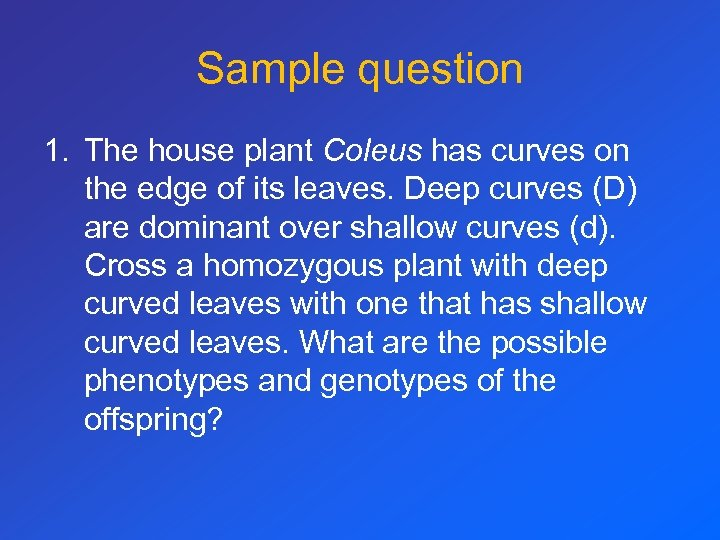Sample question 1. The house plant Coleus has curves on the edge of its leaves. Deep curves (D) are dominant over shallow curves (d). Cross a homozygous plant with deep curved leaves with one that has shallow curved leaves. What are the possible phenotypes and genotypes of the offspring?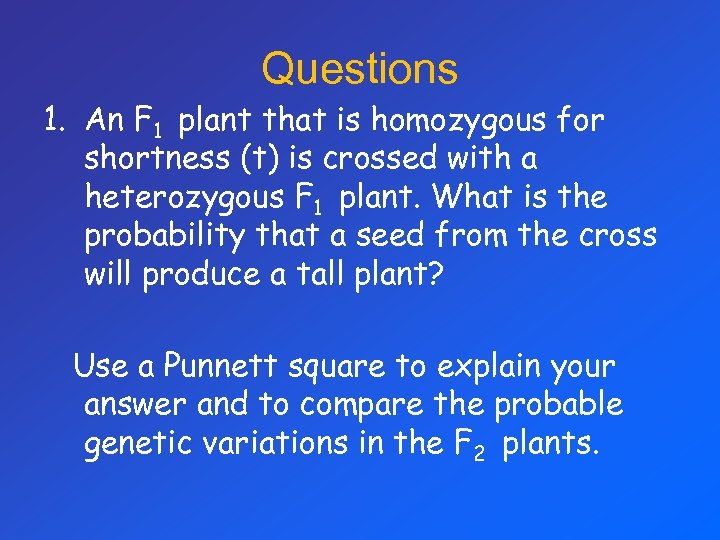Questions 1. An F 1 plant that is homozygous for shortness (t) is crossed with a heterozygous F 1 plant. What is the probability that a seed from the cross will produce a tall plant? Use a Punnett square to explain your answer and to compare the probable genetic variations in the F 2 plants.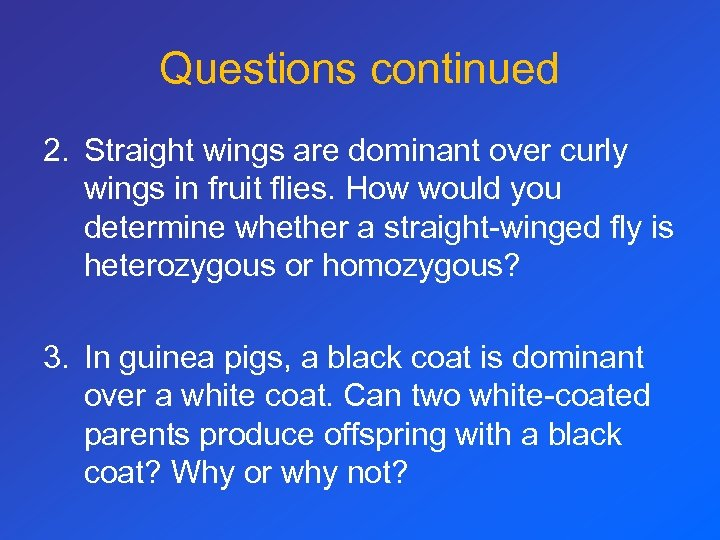Questions continued 2. Straight wings are dominant over curly wings in fruit flies. How would you determine whether a straight-winged fly is heterozygous or homozygous? 3. In guinea pigs, a black coat is dominant over a white coat. Can two white-coated parents produce offspring with a black coat? Why or why not?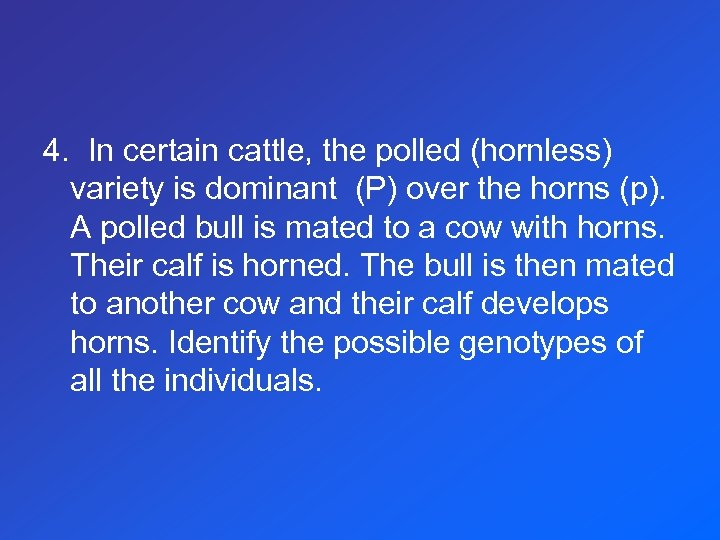4. In certain cattle, the polled (hornless) variety is dominant (P) over the horns (p). A polled bull is mated to a cow with horns. Their calf is horned. The bull is then mated to another cow and their calf develops horns. Identify the possible genotypes of all the individuals.